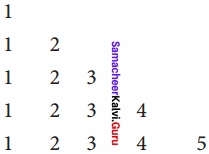# Samacheer Kalvi 12th Computer Science Solutions Chapter 6 Control Structures

Students can Download Computer Science Chapter 6 Control Structures Questions and Answers, Notes Pdf, Samacheer Kalvi 12th Computer Science Book Solutions Guide Pdf helps you to revise the complete Tamilnadu State Board New Syllabus and score more marks in your examinations.

## Tamilnadu Samacheer Kalvi 12th Computer Science Solutions Chapter 6 Control Structures

### Samacheer Kalvi 12th Computer Science Control Structures Text Book Back Questions and Answers

PART – I

12th Computer Science Chapter 6 Book Back Answers Question 1.
How many important control structures are there in Python?
(a) 3
(b) 4
(c) 5
(d) 6
(a) 3

Samacheer Kalvi Guru 12th Computer Science Question 2.
elif can be considered to be abbreviation of ……………………….
(a) Nested if
(b) If … else
(c) Else if
(d) If ……… Else
(a) Nested if

Samacheerkalvi.Guru Computer Science Question 3.
What plays a vital role in Python programming?
(a) Statements
(b) Control
(c) Structure
(d) Indentation
(d) Indentation

12th Computer Science Samacheer Kalvi Question 4.
Which statement is generally used as a placeholder?
(a) Continue
(b) Break
(c) Pass
(d) Goto
(c) Pass

Samacheer Kalvi 12th Computer Science Solutions Question 5.
The condition in the if statement should be in the form of ……………………….
(a) Arithmetic or Relational expression
(b) Arithmetic or Logical expression
(c) Relational or Logical expression
(d) Arithmetic
(c) Relational or Logical expression

Samacheer Kalvi Guru 8th Computer Science Question 6.
Which is the most comfortable loop?
(a) do..while
(b) While
(c) For
(d) if….elif
(c) For

Computer Chapter 6 Question 7.
What is the output of the following snippet?
i = 1
while True:
if i % 3 = 0:
break
print(i, end = “)
i + = 1
(a) 12
(b) 123
(c) 1234
(d) 124
(a) 12

Samacheer Kalvi Class 12 Computer Science Question 8.
What is the output of the following snippet?
T = 1
while T: print(True)
break
(a) False
(b) True
(c) 0
(d) No output
(d) No output

Class 9th Computer Questions And Answers Question 9.
Which amongst this is not a jump statement?
(a) For
(b) Goto
(c) Continue
(d) Break
(a) For

Question 10.
Which punctuation should be used in the blank?
if _
statements – block 1
else:
statements – block 2
else:
(a) ;
(b) :
(c) ::
(d) !
(b) :

PART – II

Question 1.
List the control structures in Python?

• Sequential
• Alternative or Branching
• Iterative or Looping

Question 2.
Write note on break statement?
The break statement terminates the loop containing it. Control of the program flows to the statement immediately after the body of the loop.

Question 3.
Write is the syntax of if .. else statement?
Syntax: if< condition > :
statements – block 1
else:
statements – block 2

Question 4.
Define control structure?
A program statement that causes a jump of control from one part of the program to another is called control structure or control statement. As you have already learnt in C++, these control statements are compound statements used to alter the control flow of the process or program depending on the state of the process.

Question 5.
Write a note on range() in loop?
range () generates a list of values starting from start till stop -1.
range (start.stop, [step ])
where,
start – refers to the initial value
stop – refers to the final value
step – refers to increment value, this is an optional part.

PART – III

Question 1.
Write a program to display?
A
A B
A B C
A B C D
A B C D E
Program code: for i in range(1, 6):
for i in range(65, 65 + i)
a = chr (j)
print a
print

Question 2.
Write a note on if else structure?
if .. else statement
When we need to construct a chain of the statement(s) then ‘elif’ clause can be used instead of ‘else’
Syntax:
if< condition -1 > :
statements – block 1
else:
statements – block 2

Question 3.
Using if..else..elif statement write a suitable program to display largest of 3 numbers. Display Largest of 3 Numbers?
num 1 = int (input(“Enter first number : “))
num 2 = int (input(“Enter second number : “))
num 3 = int (input(“Enter third number : “))
if (num 1 > num 2) and (num 1 > num 3):
largest = num 1
elif (num 2 > num 1) and (num 2 > num 3):
largest = num 2
else:
largest = num3
print (“The largest number is”, largest)
Output:
Enter first number: 7
Enter second number: 5
Enter third number: 4
The largest number is 7

Question 4.
Write the syntax of the while loop?
Syntax:
while< condition >
: statements block 1
[else:
statements block 2]

Question 5.
List the differences between break and continue statements?
Break statement:
Break statement has even skipped the ‘else’ part of the loop and has transferred the control to the next line following the loop block.

Continue statement:
Continue statement unlike the break statement is used to skip the remaining part of a loop and start with next iteration.

PART – IV

Question 1.
Write a detail note on for loop?
for loop:
for loop is the most comfortable loop. It is also an entry check loop. The condition is checked in the beginning and the body of the loop(statements – block 1) is executed if it is only True otherwise the loop is not executed.
Syntax:
for counter _ variable in sequence:
statements block 1
# optional block
[else:
statements block 2]
The counter_variable mentioned in the syntax is similar to the control variable that we used in the for loop of C++ and the sequence refers to the initial, final and increment value.

Usually in Python, for loop uses the rangeQ function in the sequence to specify the initial, final and increment values, ranged) generates a list of values starting from start till stop – 1.
The syntax of range O is as follows:
range (start, stop, [step])
Where,
start – refers to the initial value
stop – refers to the final value
step – refers to increment value, this is optional part.
Examples for range 0
range (1, 30, 1) will start the range of values from 1 and end at 29
range (2, 30, 2) will start the range of values from 2 and end at 28
range (30, 3, -3) will start the range of values from 30 and end at 6
range (20) will consider this value 20 as the end value(or upper limit) and starts the range count from 0 to 19 (remember always range 0 will work till stop – 1 value only).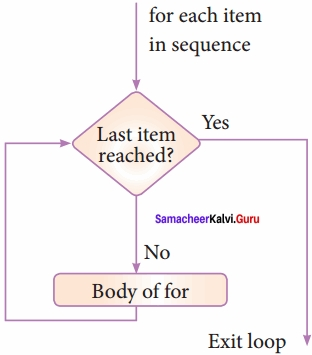# program to illustrate the use of for loop – to print single digit even number
fori in range (2, 10, 2):
print (i, end = ‘ ‘)
Output:
2 4 6 8

Question 2.
Write a detail note on if..else..elif statement with suitable example?
Nested if..elif…else statement:
When we need to construct a chain of if statement(s) then ‘elif’ clause can be used instead of ‘else’.
Syntax:
if:
statements – block 1
elif:
statements – block 2
else:
statements – block n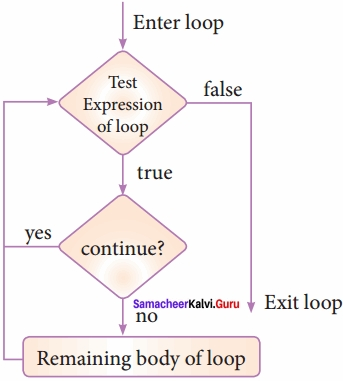In the syntax of if..elif..else mentioned above, condition – 1 is tested if it is true then statements-block 1 is executed, otherwise the control checks condition – 2, if it is true statements-block2 is executed and even if it fails statements – block n mentioned in else part is executed.

‘elif’ clause combines if.else – if.else statements to one if.elif… else, ‘elif’ can be considered to be abbreviation of ‘else if’. In an ‘if’ statement there is no limit of ‘elif’ clause that can be used, but an ‘else ’clause if used should be placed at the end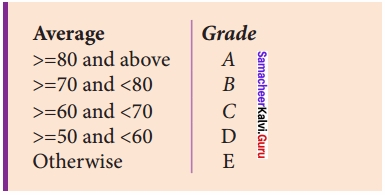m1 = int (input(“Enter mark in first subject: ”))
m2 = int (input(“Enter mark in second subject: ”))
avg = (m1 + m2)/2
if avg> = 80:
elif avg> = 70 and avg<80: print (“Grade: B”) elif avg> = 60 and avg<70: print (“Grade: C”) elif avg> = 50 and avg<60:
else:

Output 1:
Enter mark in first subject: 34
Enter mark in second subject: 78

Question 3.
Write a program to display all 3 digit odd numbers?
Coding:
lower=int(input(“Enter the lower limit for the range:”))
upper=int(input(“Enter the upper limit for the range:”))
for i in range(lower,upper+l): if(i%2!=0):
print(i,end=” “)

Output:
Enter the lower limit for the range : 100
Enter the upper limit for the range : 150
101 103 105 107 109 111 113 115 117 119 121 123 125 127 129 131 133 135 137 139 141 143 145 147 149 >>>>

Question 4.
Write a program to display multiplication table for a given number?
Multiplication table
num = int (input(“Enter the number : “))
print (“multiplication Table of “, num)
for i in range(1, 11):
print (num, “x”, i,”=”, num*i)
Output:
Enter the number: 6
Multiplication Table of 6
6 × 1 = 6
6 × 2 = 12
6 × 3 = 18
6 × 4 = 24
6 × 5 = 30
6 × 6 = 36
6 × 7 = 42
6 × 8 = 48
6 × 9 = 54
6 × 10 = 60

Practice Programs

Question 1.
Write a program to check whether the given character is a vowel or not?
ch = input(“Enter a character : “)
if ch in (‘a’, ’A’, ‘e’, ‘E’, ‘i’, ‘I’, ‘o’, ‘O’, ‘u’, ‘U’):
print (ch,’is a vowel’)
else:
print (ch the letter is not a vowel’)
Output:
Enter a character: e
e is a vowel

Question 2.
Using if..else..elif statement check smallest of three numbers?
num 1 = int (input(“Enter first number : “))
num 2 = int (input(“Enter second number : “))
num 3 = int (input(“Enter third number : “))
if (num 1 < num 2) and (num 1 < num 3):
smallest = num 1
elif (num 2 < num 1) and (num 2 < num 3): smallest = num 2 else: smallest = num 3 print(” The smallest number is”, smallest) Output: Enter first number: 12 Enter second number: 7 Enter third number: 15 The smallest number is 7 Question 3. Write a program to check if a number is Positive, Negative or zero? Answer: num = int(input(“Enter a number : “)) if num > 0:
print (“positive number”)
elif num = = 0:
print(“zero”)
else:
print (“Negative number”)
Output:
Enter a number: 2
positive number

Question 4.
Write a program to display Fibonacci series 01 1235 (up to n terms)?
n terms = int (input (“How many terms?”))
n1 = 0
n2 = 1
count = 2
# check if the number of tenns is valid
if n terms <= 0:
print (“please enter a positive integer”)
elif n terms = =1:
print (“Fibonacci sequence:”)
print(n1)
else:
print (“Fibonacci sequence :”)
print (n1, n2, end = “,”)
while count < nterms: nth = n1 + n2 print(nth, end =’, ‘) n1 = n2 n2 = nth count + = 1 Output: How many terms? 10 Fibonacci sequence: 0, 1, 1, 2, 3, 5, 8, 13, 21, 34

Question 5.
Write a program to display sum of natural numbers, upto n?
n = input(“Enter any number”) sum = 0 for i in range(i, n + 1): sum = sum + i print “sum = “, sum
Output:
Enter any number 5
sum =15

Question 6.
Write a program to check if the given number is a palindrome or not?
n = int(input(“Enter any Number : “)) temp = n rev = 0 while (n >0):
dig = n%10
rev = rev * 10 + dig
n = n // 10
if (temp = = rev):
print (“palindrome”)
else:
print (“not a palindrome”)
Output:
Enter any Number 2332
palindrome

Question 7.
Write a program to print the following pattern?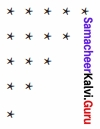Program:
for i in range(0, 5):
for j in range(5, i, -1):
print (“*”, end = “”)
print ( )

Question 8.
Write a program to check if the year is a leap year or not?
n = int (input(“Enter any year”))
if (n % 4 = 0):
print “Leap Year”
else:
print “Not a Leap Year”
Output:
Enter any Year 2000
Leap Year

### Samacheer Kalvi 12th Computer Science Control Structures Additional Questions and Answers

PART – I

Question 1.
Executable segments that yield the result are …………..
a) Operator
b) Statements
c) Keywords
d) Identifiers
b) Statements

Question 2.
A program statement that causes a jump of control from one part of the program to another is called ………………………….
Control Structure.

Question 3.
The type which we learned through alternative or branching statements is?
a) looping
b) decision making
c) functions
d) classes
b) decision making

Question 4.
A ………………………… is composed of a sequence of statements which are executed one after the another.
Sequential Statement

Question 5.
The statement in python used to transfer the control from one part of the program to another unconditionally is
a) Jump
b) loop
c) alternative
d) iterative
a) Jump

Question 6.
…………………………… is the simplest of all decision making statements.
Simple If

Question 7.
…………………………….. statement provides control to check the true block as well as the false block.
If ………. else

Question 8.
In for loop of Python, the ………….. refers to the initial, final, and increment value.
a) else
b) sequence
c) range
d) b or c
c) range

Question 9.
How many types of looping constructs are there?
(a) 1
(b) 2
(c) 3
(d) 4
(b) 2

Question 10.
The ………………….. part of while is optional.
Else

Question 11.
Control of the program flows to the statements immediately after the body of the loop by using ………… statements.
a) continue
b) pass
c) break
d) go to
c) break

Question 12.
Which one of the following is the entry check loop type?
(a) While
(b) Do while
(c) If
(d) If…else
(a) While

Question 13.
How many parameters are there in the print function?
(a) 2
(b) 3
(c) 4
(d) 5
(a) 2

Question 14.
Escape sequences can be given using …………………………. parameter in print ( ) function.
(a) Ret
(b) Let
(c) End
(d) Sep
(c) End

Question 15.
Which parameter is used to specify any special characters?
(a) Ret
(b) Let
(c) End
(d) Sep
(d) Sep

Question 16.
If the condition is checked at the beginning of the loop, then it is called as ……………………… loop.
(a) Exit
(b) Exit check
(c) Entry check
(d) Multiple
(c) Entry check

Question 17.
range ( ) generates a list of values starting from start till ………………………..
Stop – 1

Question 18.
Which is the optional part in the range( ) function?
(a) Start
(b) Stop
(c) Step
(d) Incr
(c) Step

Question 19.
The end value of the range (30, 3, -3) is ………………………..
(a) 30
(b) -3
(c) 3
(d) 6
(d) 6

Question 20.
range(20) has the range value from ……………………….. to ………………………..
0 to 19

Question 21.
range ( ) cannot take the values from ………………………..
(a) String
(b) Print
(c) List
(d) Dictionary
(b) Print

Question 22.
A loop placed within another loop is called as ………………………..
Nested loop

Question 23.
……………………….. statements are used to unconditionally transfer the control from one part of the program to another.
(a) While
(b) Jump
(c) For
(d) If
(b) Jump

Question 24.
How many keywords are there to achieve Jump statements in python?
(a) 1
(b) 2
(c) 3
(d) 4
(c) 3

Question 25.
Pick the odd one out.
break, for, continue, pass.
For

Question 26.
………………………. is used to come out of the loop.
(a) Break
(b) For
(c) Continue
(d) Pass
(a) Break

Question 27.
If a loop is left by ……………………… then the else part is not executed.
Break

Question 28.
………………………….. statement forces the next iteration to takes place.
(a) Break
(b) For
(c) Continue
(d) Pass
(c) Continue

Question 29.
……………………. is the null statement.
(a) Break
(b) For
(c) Continue
(d) Pass
(d) Pass

Question 30.
Python …………………….. will throw an error for all indentation errors.
Interpreter.

PART – II

Question 1.
What are the two types of looping construct in python?
Python provides two types of looping constructs:

1. while loop
2. for loop

Question 2.
Name the different types of alternative statements in Python?
Python provides the following types of alternative or branching statements:
Simple if statement if……else statement if….elif statement

Question 3.
From where range () takes values?
range () takes values from string, list and dictionary.

Question 4.
Give the diagram for while loop execution?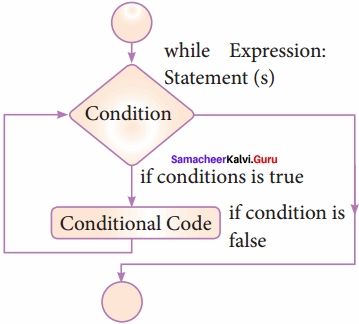Question 5.
What is the use of Jump statements in python?

• The jump statement in Python is used to unconditionally transfer the control from one part of the program to another.
• There are three keywords to achieve jump statements in Python: break, continue, pass.

Question 6.
Give the diagram for ‘for loop’ execution?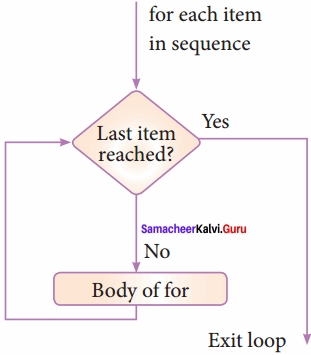Question 7.
Define nested loops?
A loop placed within another loop is called a nested loop structure. One can place a while within another while; for within another for; for within while and while within for to construct such nested loops.

Question 8.
Write a note on Jump statements in python?
The jump statement in Python is used to unconditionally transfer the control from one part of the program to another. There are three keywords to achieve jump statements in Python: break, continue, pass.

PART – III

Question 1.
Give the syntax for simple if statements and if-else
simple if
Syntax:
if:
statements – block 1
if-else
Syntax:
variable = variable 1 if condition else variable 2

Question 2.
Give the flow chart diagram for if..else statement execution?
if..else statement thus provides two possibilities and the condition determines which BLOCK is to be executed.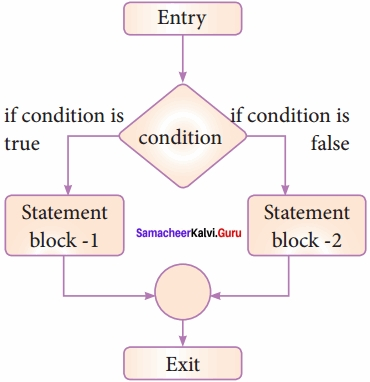Question 3.
Write a python program to check whether the given number is odd or even?
Example: #Program to check if the accepted number odd or even
a = int (input(“Enter any number:”))
if a%2 = = 0:
print (a, ” is an even number”)
else:
print (a,” is an odd number”)

Output 1:
Enter any number: 56
56 is an even number

Output 2:
Enter any number: 67
67 is an odd number

Question 4.
Give the flowchart diagram for if..elif…else statement execution?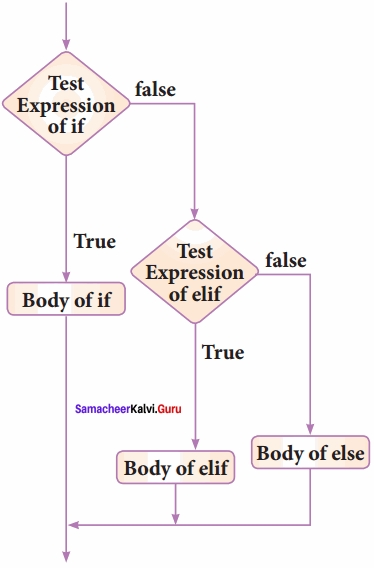Question 5.
Give a diagram to illustrate how the looping construct gets executed?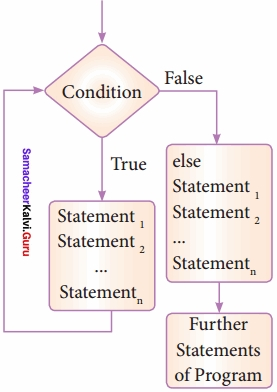Question 6.
Explain the two parameters of the print ( ) function?
print can have ended, sep as parameters, end parameter can be used when we need to give any escape sequences like ‘\t’ for tab, ‘\n’ for new-line, and so on. sep as a parameter can be used to specify any special characters like, (comma); (semicolon) as a separator between values.

Question 7.
Give the syntax for ‘for loop’?
Syntax:
for counter_variable in sequence:
statements – block 1
[else: # optional block
statements – block 2]

Question 8.
Write the program to calculate the sum of numbers from 1 to 100.
Example: # program to calculate the sum of numbers 1 to 100
n = 100
sum = 0
for counter in range (1, n + 1):
sum = sum + counter
print (“Sum of 1 until %d: %d” % (n,sum))
Output:
Sum of 1 until 100: 5050

Question 9.
Draw the flowchart to illustrate the use of break and continue statements in the loop?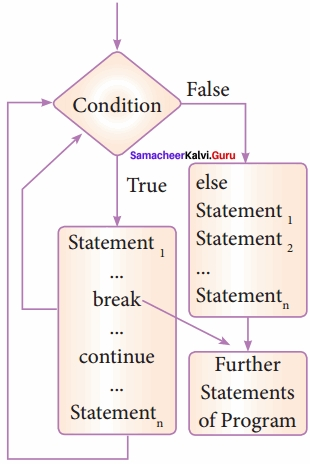Question 10.
Give the syntax for a break, continue and pass?
Syntax:
break
Syntax:
continue
Syntax:
pass

Question 11.
Write a short note on pass statements?
pass statement in Python programming is a null statement, pass statement when executed by the interpreter it is completely ignored. Nothing happens when the pass is executed, it results in no operation. pass statement can be used in the ‘if’ clause as well as within loop construct when you do not want any statements or commands within that block to be executed.

PART – IV

Question 1.
Explain If and If..else with sample programs?
Simple if statement:
Simple if is the simplest of all decision-making statements. The condition should be in the form of relational or logical expression.

Syntax:
if:
statements – block 1
In the above syntax if the condition is true statements – block 1 will be executed.

Example
# Program to check the age and print whether eligible for voting
x = int (input (“Enter your age :”))
if x > = 18:
print (“You are eligible for voting”)

Output 1:
Enter your age: 34 You are eligible for voting

Output 2:
>>>
As you can see in the second execution no output will be printed, only the Python prompt will be displayed because the program does not check the alternative process when the condition is failed.

if..else statement:
The if., else statement provides control to check the true block as well as the false block. Following is the syntax of ‘if.else’ statement.

Syntax:
if:
statements – block 1
else:
statements – block 2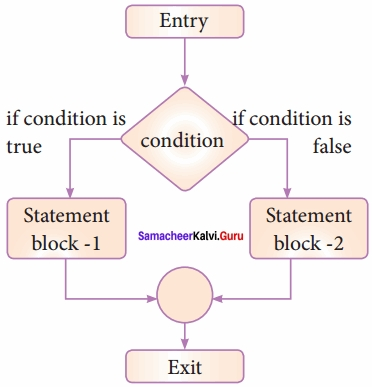if..else statement thus provides two possibilities and the condition determines which BLOCK is to be executed.
Example: #Program to check if the accepted number odd or even
a = int(input(“Enter any number :”)) if a%2==0:
print (a,” is an even number”)
else:
print (a, ” is an odd number”)

Output 1:
Enter any number: 56 56 is an even number

Output 2:
Enter any number: 67 67 is an odd number
An alternate method to rewrite the above program is also available in Python. The complete if-else can also write as:

Syntax:
variable = variable 1 if condition else variable 2

Question 2.
Explain while loop with sample program,
while loop
The syntax of while loop in Python has the following syntax:
Syntax:
while:
statements block 1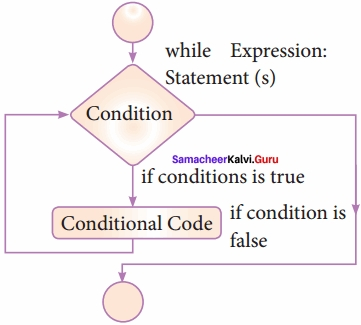In the while loop, the condition is any valid Boolean expression returning True or False. The else part of while is an optional part of while. The statements block 1 is kept executed till the condition is True. If the else part is written, it is executed when the condition is tested False.

Recall while loop belongs to entry check loop type, that is it is not executed even once if the condition is tested False in the beginning.
Example: program to illustrate the use of while loop – to print all numbers from 10 to 15
i = 10 # initializing part of the control variable
while (i< = 15): # test condition
print (i, end =’\t’) # statements – block 1
i = i + 1 # Updation of the control variable
Output:
10 11 12 13 14 15

Question 3.
Write a python program to display the following output.?